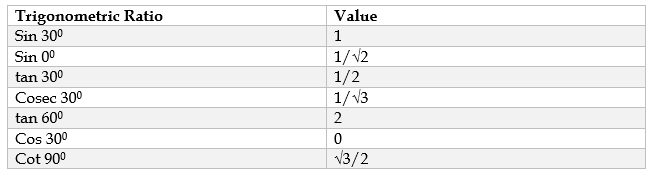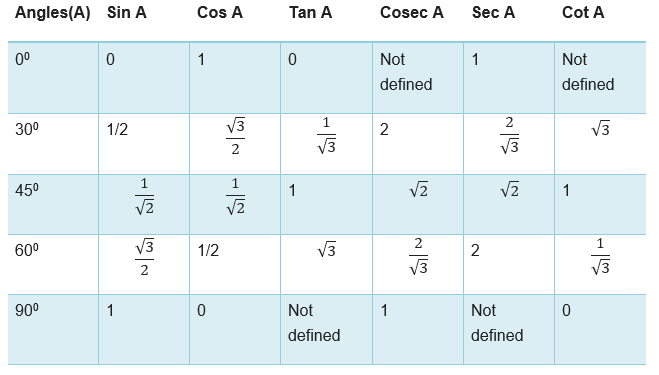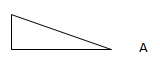# Class 10 Maths trigonometry worksheet

Given below are the Class 10 Maths trigonometry worksheet with answers.
a. Match the following
b. True and False
c. Fill in the blanks

## Match the Following

Question 1.
Match the FollowingSelf explanatory## True and False

Question 2.
True and False
a. $cos A =\frac {4}{3}$ for some angle A.
b. $tan A = \frac {sin A}{cos A}$
c. $secA =\frac {1}{cosA}$ , for an acute angle
d. $sin 60^{\circ} = 2 sin 30^{\circ}$
e. $Sin A + Cos A =1$
f. $1 + cot^2 A =cosec^2 A$
g. $sin A \times cot A= cos A$
h. $cosec 50^{\circ} = sec 40^{\circ}$

a. false
b. true
c. true
d. False
e. false
f. true
g. true
h. true

Question 3.
True and False statement
a. The value of $sin \theta + cos \theta$ is always greater than 1
b. $tan \theta$ increases faster than $sin \theta$ as θ increase
c. The value of the expression $(cos^2 23^{\circ} - sin^2 67^{\circ})$ is positive.
d. The value of the expression $(sin 80^{\circ} - cos80^{\circ})$ is negative.
e. If $cosA + cos^2 A = 1$, then $sin^2 A + sin^4 A = 1$.
f. $(tan \theta + 2) (2 tan \theta + 1) = 5 tan \theta + sec ^2 \theta$.
g. If the length of the shadow of a tower is increasing, then the angle of elevation of the sun is also increasing.
h. If a man standing on a platform 3 metres above the surface of a lake observes a cloud and its reflection in the lake, then the angle of elevation of the cloud is equal to the angle of depression of its reflection.
i. If the height of a tower and the distance of the point of observation from its foot, both, are increased by 10%, then the angle of elevation of its top remains unchanged
j. $cos^4 A - cos^2 A = sin^4 A - sin^2 A$
k. $cot^4 A - 1 = cosec^4 A - 2cosec^2 A$
l. $sin^4 A + cos^4 A = 1 - 2 sin^2 A cos^2 A$
m. $sin^4 A - cos^4 A = sin^2 A - cos^2 A = 2sin^2 A - 1 = 1 - 2 cos^2 A$

a. false
$sin 90^{\circ} + cos 90^{\circ}= 1+ 0 =1$
b. true
We know that $tan \theta = \frac {sin \theta}{cos \theta}$
Now as θ increase, sin θ increase and coθ decrease. So numerator increases and denominator decreases
In case of $sin \theta = \frac {sin \theta}{1}$
Now as θ increase, sin θ increase and denominator remains constant at 1
Hence $tan \theta$ increases faster than $sin \theta$ as θ increase

c. false
$(cos^2 23^{\circ} - sin^2 67^{\circ})$
$=(cos^2 23^{\circ} - sin^2 (90^{\circ} -23^{\circ}))$
$=(cos^2 23^{\circ} - cos^2 23^{\circ})$
=0

d. false

The value of (sin 80° - cos80°) will be positive. The value of sin80° will be very close to that of sin90° (which is 1) and similarly, the value of cos80° will be very close to that of cos90° (which is zero). Clearly, the result will be positive decimal number and should be fairly close to 0.85.

e) true

$cos A+cos^2A=1$
$cosA = 1 - cos^2A = sin^2A$
Now
$sin^2A + sin^4A$
$= Cos A+Cos^2A$
=1

f) false

g) false

h) false

i) true

j) true
$cos^4 A - cos^2 A = sin^4 A - sin^2 A$
LHS
$cos^4 A - cos^2 A\\ =cos^2 A(cos^2 A -1)\\ =-sin^2 A cos^2A\\$
RHS
$sin^4 A - sin^2 A\\ =sin^2 A(sin^2 A-1)\\ =-sin^2 A cos^2A\\$

k) true
LHS
$=cot^4A-1$
$=(cot^2A)^2-1^2$
$=(cot^2A+1)(cot^2A-1)$
Now $1+cot^2A=cosec^2A$ which implies $cot^2A=cosec^2A-1$
$=cosec^2A(cosec^2A-1-1)$
$=cosec^2A(cosec^2A-2)$
$=cosec^4A-2cosec^2A$
=RHS

l) true
LHS
$sin^4 A + cos^4 A 2sin^2 A cos^2 A$
$=sin^4 A + cos^4 A + 2sin^2 A cos^2 A - 2sin^2 A cos^2 A$
$=(sin^2 A + cos^2 A)^2 - 2sin^2 A cos^2 A$
$=1- 2sin^2 A cos^2 A$

m) true
$sin^4 A - cos^4 A$
=$(sin^2 A + cos^2A)(sin^2 A - cos^2 A)$
Now as $sin^2 A + cos^2 A =1$
$=sin^2 A - cos^2 A$
$=sin^2 A - (1- sin^2 A)$
$=(2sin^2 A - 1 )$

## Fill in the blanks

Question 4
Fill in the blanks:
a. $5 cos 0^{\circ} + sin 90^{\circ}$ = __________
b. $tan 0^{\circ} =$ _____________
c. $tan 90^{\circ}$ is _____________
d. If $sin A= 1$, then A = ________________
e. $2 sin^2 60^{\circ}$ = ___________
f. $2 cos^2 45^{\circ}$ = __________________
g. $sin^2 A + cos^2 A=$ _______________
h. $(1 + tan^2 A ) (1 + sinA ) (1 - sin A) =$ ________.

a. 6
b. 0
c. undefined
d. 90
e. 3
f. 1
g. 1
h. 1

Question 5.
Write the values cos 0°, cos 45°, cos 60° and cos 90°. What happens to the values of cos as angle increases from 0° to 90°?

$\cos 0^{\circ}= 1$
$\cos 45^{\circ}= \frac {1}{\sqrt {2}}$
$\cos 60^{\circ}= \frac {1}{2}$
$\cos 90^{\circ}= 0$
We can see that values of cos decreases as angle increases from 0° to 90°

Question 6.
Write the values of sin 0°, sin 30°, sin 45°, sin 60° and sin 90°. What happens to the values of sin as angle increases from 0° to 90°?

$\sin 0^{\circ}= 0$
$\sin 30^{\circ}= \frac {1}{2}$
$\sin 45^{\circ}= \frac {1}{\sqrt {2}}$
$\sin 60^{\circ}= \frac {\sqrt {3}}{2}$
$\sin 90^{\circ}= 1$
We can see that values of sin increases as angle increases from 0° to 90°

Question 7.
Write the values of tan 0°,tan 30°, tan 45°, tan 60° and tan 90°. What happens to the values of tan as angle increases from 0° to 90°?

$\tan 0^{\circ}= 0$
$\tan 30^{\circ}= \frac {1}{\sqrt {3}}$
$\tan 45^{\circ}= 1$
$\tan 60^{\circ}= \sqrt {3}$
$\tan 90^{\circ}= undefined$
We can see that values of tan increases as angle increases from 0° to 90°

Question 8.
If $\sin A =\frac {3}{5}$ .find cos A and tan A.

Given $\sin A= \frac{3}{5}$
Or $\frac {P}{H}=\frac{3}{5}$
Let P=3k and H=5kNow By Pythagoras theorem
$P^2 + B^2 =H^2$
$9k^2 + B^2 =25k^2$
Or $B =4k$
Now $\Cos A = {B \over H} = \frac {4}{5}$
$\tan A = {{\sin A} \over {\cos A}} = \frac {3}{4}$

Question 9.
If $cosec A = \sqrt {10}$. find other five trigonometric ratios.

Given $cosec A = \sqrt {10}$
Or $\frac {H}{P}=\sqrt {10}$
Let $H=\sqrt {10} k$ and P=kNow By Pythagoras theorem
$P^2 + B^2 =H^2$
$k^2 + B^2 = 10k^2$ B= 3k
Now $sinA = {P \over H} = \frac {1}{\sqrt {10}}$
$cosA = {B \over H} = \frac {3}{\sqrt {10}}$
$tan A = {{SinA} \over {CosA}} = \frac {1}{3}$
$sec A = \frac {1}{cos A} = \frac {\sqrt {10}}{3}$
$cot A = {{cosA} \over {sinA}} = 3$

Question 10.
In a right triangle ABC right angled at B if $sin A =\frac {3}{5}$ . find all the six trigonometric ratios of C.

Given $sin A= \frac{3}{5}$
Or $\frac {P}{H}=\frac{3}{5}$
Let P=3k and H=5kNow By Pythagoras theorem
$P^2 + B^2 =H^2$
$9k^2 + B^2 =25k^2$
Or $B =4k$
Now $CosA = {B \over H} = \frac {4}{5}$
$tan A = {{SinA} \over {CosA}} = \frac {3}{4}$
$cot A = {{cosA} \over {sinA}} = \frac {4}{3}$
$cosec A = {{1} \over {sinA}} = \frac {5}{3}$
$sec A = {{1} \over {cos A}} = \frac {5}{4}$

Now A + B + C=180
C=90 -A
$sin C = sin (90 -A) = cos A = \frac {4}{5}$
$cos C = cos (90 -A) = sin A = \frac {3}{5}$
$tan C = tan (90 -A) = cot A = \frac {4}{3}$
$cosec C = cosec (90 -A) = sec A = \frac {5}{4}$
$sec C = sec (90 -A) = cosec A = \frac {5}{3}$
$tan C = tan (90 -A) = cot A = \frac {4}{3}$

Question 11.
The value of $(sin30 ^{\circ} + cos30^{\circ}) - (sin60^{\circ} + cos60^{\circ})$ is

$(sin30 ^{\circ} + cos30^{\circ}) - (sin60^{\circ} + cos60^{\circ})$
$=(\frac {1}{2} + \frac {\sqrt {3}}{2}) - (\frac {\sqrt {3}}{2} + \frac {1}{2})$
=0

Question 12.
If $sin B =\frac {1}{2}$ , show that $3 cos B - 4 cos^3 B = 0$

$sin B =\frac {1}{2}$
So $\angle B =30 ^{\circ}$
$cos B = cos 30 =\frac {\sqrt {3}}{2}$
Now
$3 cos B - 4 cos^3 B$
$=3 \frac {\sqrt {3}}{2} - 4 (\frac {\sqrt {3}}{2})^3$
$=\frac {3\sqrt {3}}{2} -\frac {3\sqrt {3}}{2}$
=0

Question 13.
If $tanA + \frac {1}{tanA}= 2$, find the value of $tan^2 A + \frac {1}{tan^2 A}$

$tanA + \frac {1}{tanA}= 2$
Squaring both the sides
$tan^2 A + \frac {1}{tan^2 A} + 2 = 4$
$tan^2 A + \frac {1}{tan^2 A}=2$

Question 14. Evaluate the following:
$2 sin^2 30^{\circ} - 3cos^2 45^{\circ} + tan^2 60^{\circ}$

$2 sin^2 30^{\circ} - 3cos^2 45^{\circ} + tan^2 60^{\circ}$
$=2 (\frac {1}{2})^2 - 3 (\frac {1}{\sqrt {2}})^2 + (\sqrt {3})^2$
$=\frac {1}{2} - \frac {3}{2} + 3$
=2

Question 15. Evaluate:
$sin^2 30^{\circ} cos^2 45^{\circ} + 4tan^2 30^{\circ} + \frac {1}{2} sin^2 90^{\circ} - 2 cos^2 90^{\circ} + \frac {1}{24}cos^2 0^{\circ}$

$sin^2 30^{\circ} cos^2 45^{\circ} + 4tan^2 30^{\circ} + \frac {1}{2} sin^2 90^{\circ} - 2 cos^2 90^{\circ} + \frac {1}{24}cos^2 0^{\circ}$
$= \frac {1}{4} \times \frac {1}{2} + 4 \times \frac {1}{3} + \frac {1}{2} -0 + \frac {1}{24}$
$= \frac {1}{8} + \frac {4}{3} + \frac {1}{2}+ \frac {1}{24}$
$=\frac {3+ 32 + 12 +1}{24}$
$=2$

Question 16. Evaluate:
$cot^2 30^{\circ} - 2 cos^2 60^{\circ} - \frac {3}{4} sec^2 45^{\circ} - 4sec^2 30^{\circ}$

$cot^2 30^{\circ} - 2 cos^2 60^{\circ} - \frac {3}{4} sec^2 45^{\circ} - 4sec^2 30^{\circ}$
$= 3 - \frac {1}{2} -3 -4 \times \frac {4}{3}$
$= \frac {-13}{3}$

Question 17. Prove that:
$cos 1^{\circ}.cos 2^{\circ} .cos3^{\circ} .... cos 180^{\circ} = 0$

Now as $cos 90^{\circ}=0$
$cos 1^{\circ}.cos 2^{\circ} .cos3^{\circ} .... cos 180^{\circ}=0$

Question 18
Prove the following identity
$(sin A - cosec A)^2 + (cos A - sec A)^2 = tan^2 A+cot^2 A-1$
Question 19
Prove the following identity
(i) $cos^6 A + sin^6 A = 1 - 3 sin^2 A Cos^2 A$
(ii)$\frac {1}{cot A + tan A} = sin A cos A$
Question 20
Prove the following
(i) $(sin A + cos A)(cot A + tan A)=sec A + cosec A$
(ii)$sin 30 cos 60 + sin 60 cos 30=1$

## Summary

This Class 10 trigonometry worksheet with answers is prepared keeping in mind the latest syllabus of CBSE . This has been designed in a way to improve the academic performance of the students. If you find mistakes , please do provide the feedback on the mail.You can download in PDF form also using the below linksGo back to Class 10 Main Page using below links

### Practice Question

Question 1 What is $1 - \sqrt {3}$ ?
A) Non terminating repeating
B) Non terminating non repeating
C) Terminating
D) None of the above
Question 2 The volume of the largest right circular cone that can be cut out from a cube of edge 4.2 cm is?
A) 19.4 cm3
B) 12 cm3
C) 78.6 cm3
D) 58.2 cm3
Question 3 The sum of the first three terms of an AP is 33. If the product of the first and the third term exceeds the second term by 29, the AP is ?
A) 2 ,21,11
B) 1,10,19
C) -1 ,8,17
D) 2 ,11,20